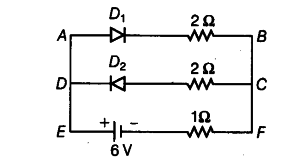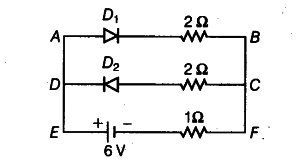# Assuming that the two diodes used in the electric circuit

Assuming that the two diodes used in the electric circuit as shown in the figure are ideal, find out the value of the current flowing through 1 Ω resistor.According to the question,Equivalent resistance, { R }_{ AB } = 2 + 1 = 3Ω
1/R’ = 1/2 + 1/3 = 3+2/6 = 5/6 Ω
R’ = 6/5 Ω
{ I}_{ EF } = V/R’ = 6/6/5 = 5A